Diploma In ICT Module 2 Visual Programming  2016

Exam Year:2016

Exam code:2920-205Information communication technology past  papers

DIPLOMA IN INFORMATION COMMUNICATION TECHNOLOGY
MODULE II
VISUAL PROGRAMMING
3 Hours
Instructions to candidates
This paper consists of eight questions.
Answer any five of the eight questions.
All questions carry equal marks.

1. (a ) Outline four events associated with a VScroll control in a Visual Basic programming language. ( 2 mark)
(b) (i) Explain the function of ADO Recordset Object as used in Visual Basic programming language. (2 marks)
(ii) Outline four functions available in the RptFunction control of a Visual Basic program language.( 4 marks)

(c ) Distinguish between Unload Me and Form1.Hide as used in a Visual Basic Programming language. ( 4 marks)

(d) Write a Visual Basic program that repeatedly accepts a non-zero numeric value from a user and store it in a dynamic array named array 1. The program should then display the values in a list box and terminates when a user enters a zero value.
Attach the code to a command button. Use do while loop. ( 8 marks)

2. (a ) Outline the functions of each of the following elements of Integrated Development Environment (IDE) in a Visual Basic Program.
(i) Project form ( 1 mark)
(ii) Menu bar ( 1 mark)
(iii) Tool bar ( 1 mark)
(iv) Code window. ( 1 mark)
(b) Interpret the following Visual Basic program code.( 5 marks)
Public Sub SubFrmCntr
With Form 1
.Top = (Screen.Height – .Height) / 2
.Left = (Screen.Width – .Width) / 2
End With
End Sub

(c ) Write a Visual Basic program that allows a user to enter the strings ‘Alex’ and 1234 into respective text boxes in a login screen.
If the two are valid, a form named frmain is opened, otherwise a message ‘invalid name or password’ is displayed on a message box. Attach the code to a command button. ( 5 marks)

(d) Asha created a Visual Basic program interface for her application.
Explain three modes through which she could set the properties for the controls included in the interface. ( 6 marks)

3. (a) Define each of the following terms as used in a Visual Basic Programming language:
(i) Form module ( 1 mark)
(ii) Application (1 mark)
(iii) Project. ( 1 mark)
(b) (i) Explain a challenge that companies with applications built using Visual Basic 6.0 compiler may have with the changing software technology. ( 2 marks)
(ii)Outline four limitations of using Visual Programming languages. ( 4 marks)

(c ) (i) List two database objects in Visual Basic other than ADO that may be used to manipulate a database file. ( 2 marks)
(ii) Distinguish between method and a function as used in a Visual Basic Programming language. ( 4 marks)

(d) Write a Visual Basic program that displays the sequence of numbers; 50, 45,40,50,…0 on a listbox together with their cumulative sum.
Use while…wend loop.
Attach the code to a command button. ( 5 marks)

4. (a) A programmer was advised to decompose a large module into multiple procedures.
Explain two reasons for this. ( 4 marks)
(b) Write a Visual Basic Program statement that could be used to perform each of the following:
(i) display an image named bitmap.gif using image control; ( 2 marks)
(ii) display the string “Hello Kenya….” On a label in a second form from the first form; ( 2 marks)
(iii)position a shape at centre horizontally on the screen. ( 2 marks)

(c ) (i) In a Visual Basic program, an array named Sales stores 10 decimal values.
Write a statement that resizes the array to 50 elements while retaining the previous values. ( 2 marks)
(ii) Assume that a procedure has the following array declaration:
Const x As Integer = 30
Dim intPoints (x) As Interger
Write a For…Next loop segment of the code that add each element of the array into a listbox control. ( 2 marks)

(d) An international;company dealing with beauty products pays a commission to the sales workers based on the criteria shown in table 1. Use it to answer the question that follows:
Sales Volume (\$)———Commission
5000 and over————15 %
4000-4999—————-12 %
3000-3999—————-9 %
2000-2999—————-6 %
Less than 2000———–3 %
Table 1

Write a Visual Basic program that allows a user to enter the name of the sales agent and the sales volume made using text boxes.
The program should then compute the commission and output the sales agent and the commission in a picture box. Use select case control structure and attach the code to a command button. ( 6 marks)

5. (a) Explain three types of code stepping features used in a Visual Basic Programming language. ( 6 marks)
(b) With the aid of an example, explain the purpose of library functions in a Visual Basic Programing language. ( 3 marks)
(c ) Distinguish between Datafield and DataSource properties in Visual Basic. ( 4 marks)
(d) The future value (FV) of a property worth PV appreciating at the rate of R% per annum for a period of T years is given by the formula: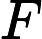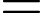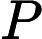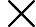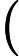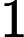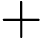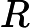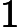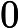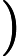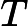Write a Visual Basic program that uses a function to calculate FV when a user enters the values of PV, R and T in text boxes. The program should then display the results in a message box in a currency format.
Attach the main procedure to a command button. ( 7 marks)

6. (a) Explain the function of each of the following sections of a Visual Basic DataReport:
(i)report footer( 2 marks)
(ii) page footer. ( 2 marks)

(b) Outline the function of each of the following Visual Basic database tools
(i) Data Environment ( 1 mark)
(ii) Data View ( 1 mark)
(iii)Data Project ( 1 mark)
(iv) Visual Data Manager. ( 1 mark)

(c ) Figure 1 shows a Visual Basic program form designed to enter data using a ADO control to a database file having the fields named Gender, English Kiswahili and French.
Use it to answer the question that follows.

Write a procedure attached to a command button that will achieve this.
(Assume that all the properties associated with Adodc 1 control have been set) ( 7 marks)(d) A student created the following Visual Basic Program to determine the roots of quadratic equations.
Use it to answer the question that follows.

Private Sub Command1_Click()
Dim a, b, c, d, x1, x2

a = Val(Text1.Text)
b = Val(Text2.Text)
c = Val(Text3.Text)

d = (b ^ 2 – 4 * a * c)

x1 = (-b + Sqrt(d)) / (2 * a)
x2 = (-b – Sqrt(d)) / (2 * a)

Text4.Text = Str(x1)
Text5.Text = Str(x2)

End Sub

Rewrite the program to include an error handler with error code 5 and 6 to test for the division by zero and square root of a negative number respectively .
When activated, the error handler displays a more descriptive error message. ( 5 marks)

7. (a) Explain the function of each of the following properties as used in Visual Basic Program screen design:
(i) Anchor ( 2 marks)
(ii) Dock. ( 2 marks)
(b) Outline four access rights to user that can be set using cursor mode property of ADO
Data control. ( 4 marks)
(c ) (i) State the order of precedence when solving the following expression in Visual Basic Programming language:

(A * B) / C ^ D – E + F ^ G

(ii)Distinguish between \and mod operators as used in Visual Basic Programming. ( 4 Marks)
(d) The body mass index (BMI) of a person is computed based on the body in kilograms and the body height in meters using the formulaWrite a Visual Basic Program that allows a user to enter the weight and the height in text boxes.
Using a function, the program should compute the BMI.
The results should be displayed on a label formatted to 2 decimal places.
Attach the code to a command button. ( 6 marks)

8. (a) Tindy, a Visual Programming student had problems with a program she was writing.
Explain one way that would show that her program had each of the following errors:
(i) Compilation (2 marks)
(ii) Run time. ( 2 marks)
(b) Distinguish between Visual Basic array statements declared in (i) and (ii) ( 4 marks)
(i) Public sub command1_click()
DIM Customers (200) As String

(ii)Private sub command1_click()
Option Base 1
DIM Customers (200) As String

(c ) State the difference between conditional and unconditional loop as used in programming.( 4 marks)

(d) Write Visual Basic program that allows a user to enter 10 integer values into an array.
The program should then sort the values using bubble sort technique and display the result in a picturebox.
Attach a code to a command button.( 8 marks)